This poster was presented as Poster #195 at the Fifth International Conference on Functional Mapping of the Human Mapping (HBM'99) held in D³sseldorf, Germany, June 22-26, 1999.

The abstract for this poster was published in NeuroImage, vol. 9, part 2, pg. S195, 1999.

Abstract

GENERATING CONFORMAL FLAT MAPS OF THE
CORTICAL SURFACE VIA CIRCLE PACKING

1Monica K. Hurdal, 1De Witt L. Sumners, 2Ken Stephenson,
1Philip L. Bowers, 3,4David A. Rottenberg1Department of Mathematics, Florida State University, Tallahassee, FL, U.S.A. 2Department of Mathematics, University of Tennessee, Knoxville, TN, U.S.A. 3PET Imaging Center, VA Medical Center, Minneapolis, MN, U.S.A. 4Department of Radiology, University of Minnesota, Minneapolis, MN, U.S.A. 1,2,3,4Members of the International Neuroimaging Consortium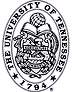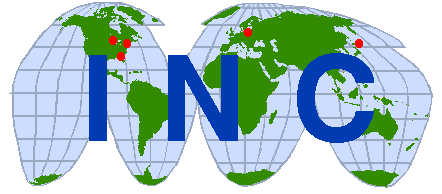Abstract a circle packing is a configuration of circles with a specified pattern of tangencies We present a novel method which uses circle packings to create flat maps of the cortical surface. these maps exhibit conformal behavior in that angular distortion is controlled
 Surface Flattening it is impossible to flatten a curved 3D surface without introducing linear and areal distortion  a surface can be flattened without introducing angular distortion: a conformal flat map  a piecewise flat bounded surface which is simply connected has no holes and no handles (ie. a 2D disk) a triangulated representation of a cortical surface is topologically equivalent to a disk
 Riemann Mapping Theorem (1854) If D is any simply-connected open set on a surface, with a distignuished point a an element of D and a specified direction (tangent vector) through a, then there is a UNIQUE conformal map (1-1 bijection) which takes D to the interior of the unit circle O in the plane, with a --> 0 and the specified direction point in the positive X direction  (see Figure 1). CONFORMAL MAPPINGS EXIST!
 Circle Packing let K represent any simply-connected triangulated surface represent each vertex, v, of K by a circle cv such that each vertex is located at the center of its circle if vertices v and w are connected by an edge, then the circles cv and cw are tangent assign a positive number to each boundary vertex this gives a circle packing for K (see Figure 2)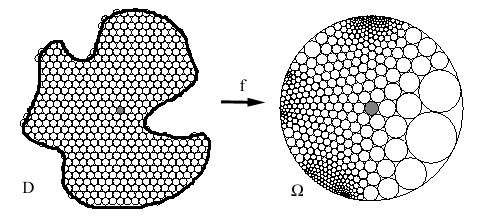Figure 1: Example of a conformal map.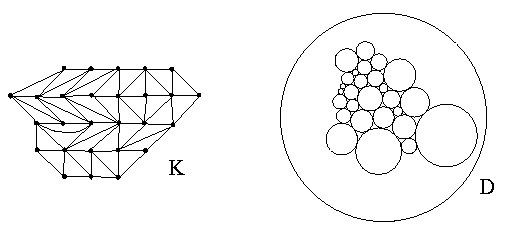Figure 2: A triangulation (left figure) and its resulting circle packing (right figure).
 Circle Packing Algorithm a new algorithm which computes the circle packing of a simply connected triangulated surface result is an approximation to unique discrete conformal maps from a 3D cortical surface to the Euclidean plane, the hyperbolic plane or the 2-sphere to our Euclidean eyes, portions of the hyperbolic map near the origin have little distortion and regions near the map border are greatly distorted (Figure 3)
 it is possible to move the focus of the hyperbolic map to change the regions which are in focus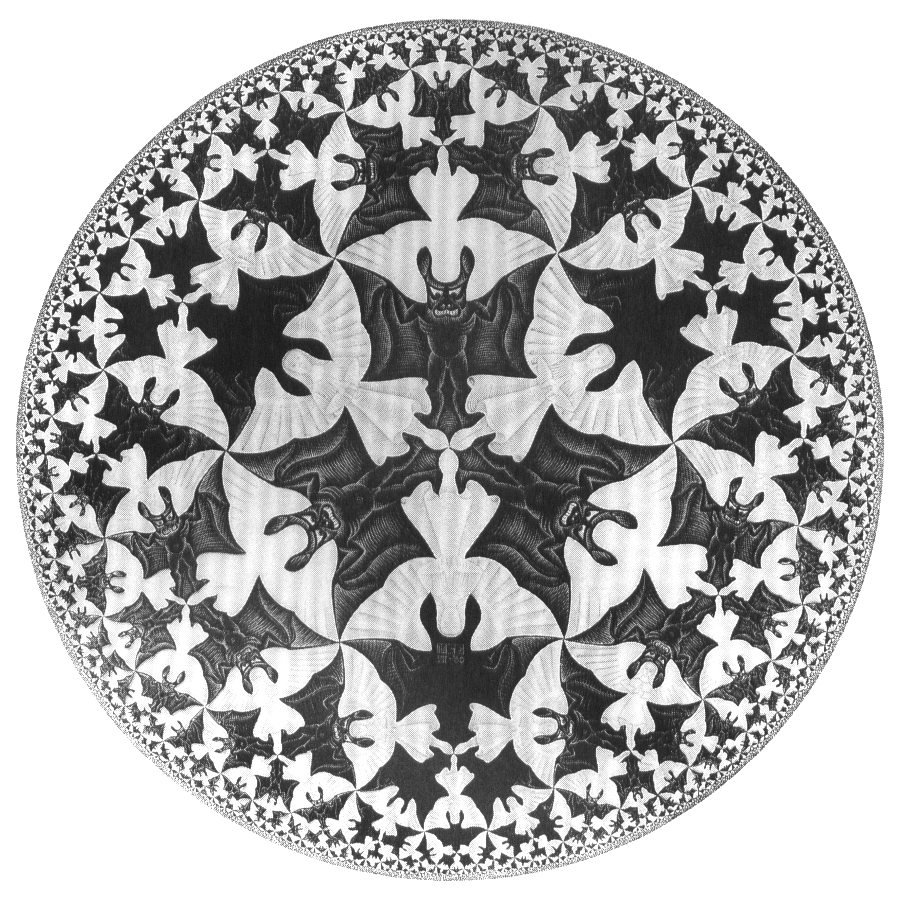Figure 3: Planar representation of the hyperbolic plane . Each devil is the same hyperbolic area. Since we look at this map with Euclidean eyes, regions near the boundary of the hyperbolic map appear greatly distorted.
 uniqueness of the conformal map is guaranteed by choosing 2 points (ie. anatomical landmarks) or 1 point and a direction on the cortical surface see Figures 4, 5 and 6 for examples of quasi-conformal flat maps created using circle packing on data from the Macaque monkey see Poster #194 for examples of quasi-conformal flat maps in the Euclidean plane, the hyperbolic plane and on a sphere using data from the human cerebellumFigure 4: Triangulated surface representing the left hemisphere of the Macaque monkey . Colors correspond to the following cortical regions: pink = occipital lobe; light green = parietal lobe; blue = temporal lobe; orange = frontal lobe; purple = limbic cortex; bright green = extra cuts introduced by Drury et al.  to use as part of the boundary for flat maps.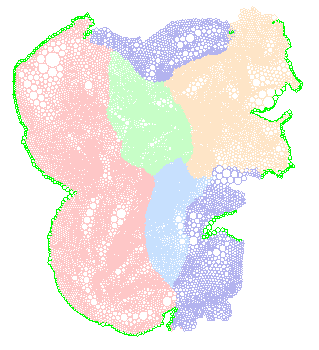Figure 5: Quasi-conformal Euclidean flat map of Macaque hemisphere. Colors correspond to regions shown in Figure 4.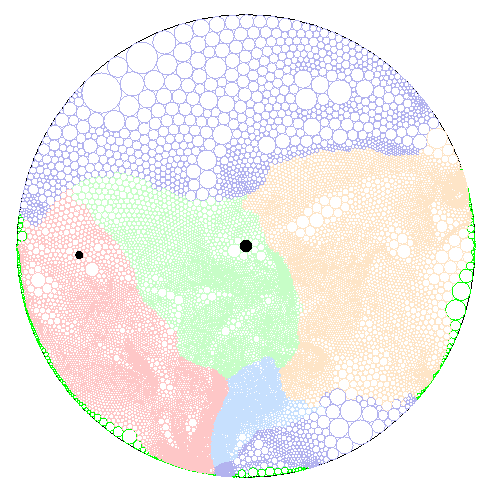Figure 6: Quasi-conformal hyperbolic flat maps of the Macaque hemisphere. Colors correspond to regions shown in Figure 4. The origin (map focus) is marked in black in the center of the maps. An alternate focus (marked in black on the left of the left map) is used as the focus for the right map. The focus from the left map is marked in black on the right side of the right map.
 The Algorithm: Case 1 a piecewise flat surface composed of equilateral triangles, with sides of length L each vertex, v, has the conditions described for a circle packing each vertex, v, is represented in the plane by a circle, cv, with radius L/2 a vertex, v, belongs to k triangle faces, giving k angles at v the angle sum,(v), is defined to be the sum of these angles about v
 a set of circles can be "flattened" so they fit together in the plane if(v) = 2 Pi curvature is concentrated at the interior vertices to flatten the surface (see Figures 7 and 8) when(v) < 2 Pi there is positive curvature (cone point) which is represented by a gap between the circles around v, so shrink the circles around v until all the circles around v are tangent when(v) = 2 Pi there is zero curvature and the map is already flat at v
 when(v) > 2 Pi there is negative curvature (saddle point) which is represented by overlapping circles in the plane, so expand the circles around v until all circles around v are tangent iteratively repeat this procedure at each vertex to obtain a circle packing this circle packing is guaranteed to be unique (up to certain transformations) and quasi-conformal (bounded amount of angular distortion) by the Circle Packing Theorem and the Ring Lemma 
 denote t as the Euclidean measure of an angle at vertex v in the 3D surface conformal flattening preserves the proportion of t in the flattened surface the angular image of t has Euclidean measure 2*Pi*t/(v) to reduce the angular distortion to zero refine the triangles in the surface by subdividing each triangle into smaller triangles and repeat the above procedure until the map converges to the unique conformal mapFigure 7: Example of how positive curvature (left) and negative curvature (right) are represented in the plane and how the circles need to be adjusted to achieve a circle packing (middle).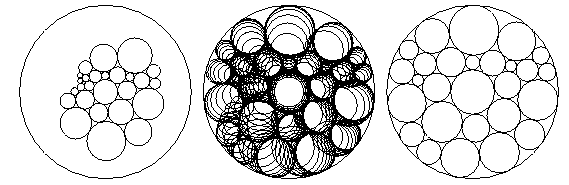Figure 8: Example illustrating how a circle packing (left) is iteratively adjusted (middle) to produce a quasi-conformal circle packing (right).
 The Algorithm: Case 2 surface triangulation is not equilateral modified circle packing algorithm where each vertex, v, is represented in the plane by a circle, cv if vertices v and w are connected by an edge, then the distance between circles cv and cw is given by the inversive distance (a conformal invariant of circle pairs) between v and w inversive distance rather than circle tangency is preserved
 Advantages and Benefits a conformal flat map is mathematically unique a unique conformal surface coordinate system can be installed on the flat map by choosing 2 points or a point and a direction no cuts required on cortical surface to enhance flattening hyperbolic maps allow the map focus and distortion to be transformed and changed minimal computation times on a 400MHz PII PC (minutes for the human cerebellum)
 For more information... on the applications of this software, see our poster "A Quasi-Conformal Flat Map of the Cerebellar Cortex" (poster #194) for a demonstration of the software used to create these flat maps, see our poster "CirclePack: Software for Creating Quasi-Conformal Flat Maps of the Brain" (poster #250) contact: Dr. Monica K. Hurdal, Department of Mathematics, Florida State University, Tallahassee, FL., U.S.A., 32306-4510. Phone: (+1-850) 644-7378; Email: mhurdal@math.fsu.edu; WWW: http://www.math.fsu.edu/~mhurdal or visit the International Neuroimaging Consortium page ( http://pet.med.va.gov:8080/incweb/). This work has been supported in part by NIH grants MH57180 and NS33718.
 References  Polya, G., Mathematical Discovery, Volume 2, John Wiley & Sons, New York, 1968.  Ahlfors, L.V., Complex Analysis, McGraw-Hill Book Company, New York, 1996.  Schattschneider, D., Visions of Symmetry, Notebooks, Periodic Drawings and Related Work of M.C. Esher, 1990.  Drury, H. A., et al., J. Cog. Neurosci., 1996, 8:1-28.  Rodin, B., Sullivan, D., J. Differential Geometery, 1987, 26:349-360.  Bowers, P., Stephenson, K., Uniformizing Dessins and Belyi Maps via CirclePacking, Submitted to Trans. A.M.S. and available at http://www.math.fsu.edu/~bowers

Updated July 1999.